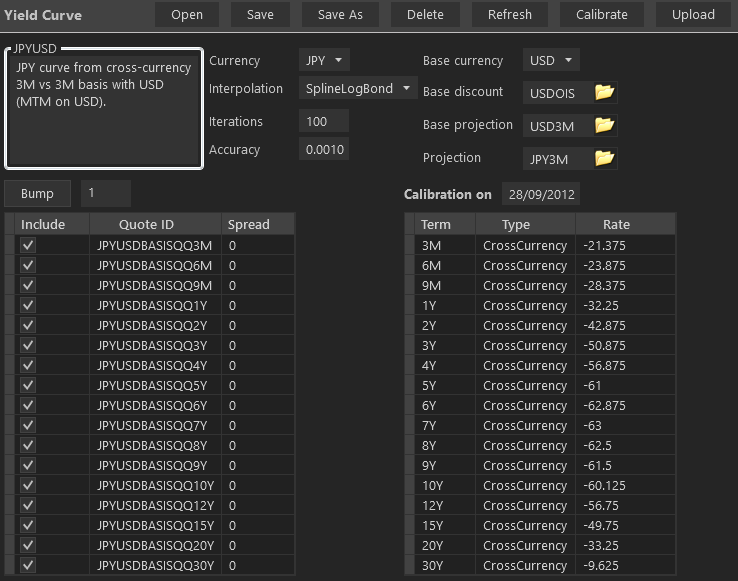Yield Curves

Yield curve construction is at the core of the pricing of virtually all trades and is rather complex to configure. As for most objects it is best to create a new curve by copying an existing one (i.e. doing a ‘Save As’), but it may not be easy to know which existing curve is of a type that is closest to the desired one.

#### Dependencies and Types

We recommend an approach based on dependencies, as some curves depend on other curves which depend on other curves, etc… The curves should be set in the following order, corresponding to both types and dependency structures

1. OIS: these curves have 0 dependencies, i.e. are constructed from the knowledge of the OIS rates only
2. Libor: these curves typically have 1 dependency, the OIS curve in the same currency, assuming the Libor swaps are collateralized.
3. Tenor: these curves typically have 2 dependencies, the OIS and the base curves. Say that you want to create the JPY 3M curve. You will have to create first the JPY OIS, then the JPY 6M that depends on JPY OIS, and only then can you build the JPY 3M curve out of the 3M vs 6M tenor basis quotes.
4. Cross-Currency: these curves usually have 3 dependencies in an explicit manner, but may have more implicitly. Being constructed either from cross-currency basis or cross-currency swap rates, they are explicitly dependent on the OIS and Libor curve in the base currency, and potentially on the Libor curve in the foreign (implied) currency. However, there may be more dependencies due to the dependencies of the dependents. In the example of the JPYUSD curve (i.e. the JPY cross-currency curve implied form USD/JPY cross-currency swaps), the constructed curve has dependencies on: JPY OIS, JPY 6M, JPY 3M, USD OIS, USD 3M.

Note that the above represents a simplified view only. In practice there are more types of underlying quotes and dependency patterns:

• Libor curves often have their short ends built from deposit rates, futures and/or FRAs.
• Tenor curves may be built from swap rates at some maturity pillars and tenor basis at other pillars. They may also be built from swap rate spreads. At the short ends they can be built from the same types of rates as the Libor curves.
• Cross-currency curves are typically built from cross-currency basis or cross-currency swap rate quotes on the mid to long ends, but the short ends may be built from FX forwards.

#### CSA Curves

CSA curves in our library are what we call curves that are not obtained directly from quotes but instead using the ‘forward FX invariance’, i.e. the assumption that the forward FX rate is independent of the collateralization. This assumption makes it possible to obtain discount factors in cross-currency situations where there are no quotes available in the market to imply a curve.

Take the example of USD cash-flows collateralized in JPY. These flows should be discounted with a USD curve with JPY basis. Such a curve should be built from quotes of cross-currency USD/JPY swaps collateralized in JPY, such that we would be able to imply a USD discount curve. Unfortunately such quotes do not exist (to our knowledge), and thus the needed curve is in principle unknown.

Assuming the forward FX invariance means that we can transform the USD cash-flows into JPY ones using the forward FX, then discount the resulting JPY flows with JPY OIS because the collateral is in JPY. The resulting discount factors make up the USDJPY curve, that is, the curve to discount USD flows collateralized in JPY.

#### Cheapest-To-Deliver Curves (CTD)

CTD curves are a simplified way of pricing in the optionality due to the choice of collateral currency. The basic principle underlying the construction is to create a grid of time intervals (ideally daily but more realistically larger) and on each interval to choose the forward rate to be the optimum one among the eligible discounting curves. The whole discount curve is then backed out from its forwards.

#### Creating a curve

Taking the example of a cross-currency curve, the configuration screen will typically look likeThe fields Currency, Interpolation, Iterations and Accuracy are common to most curves (except CSA and CTD). The Interpolation field is a string representing both the type of interpolation and the interpolated quantity (bond, log-bond, zero-rate). The Iterations field is the maximum number of iterations of the optimization algorithm, and the field Accuracy is the accuracy (in basis points) where the optimization will stop.

The other typical inputs are the market rates in the left datagrid, which can be chosen with right-click of the mouse. Of course, these market rates must have been created previously for them to be available as choices. Market rates are created from Market->Quotes->Interest Rates.

The remaining inputs are specific to the cross-currency curves, in that they specify the dependency structure.